# College Physics

Physics & Astronomy

## Quiz 1 : Electric Forces and Electric FieldsLooking for Introductory Physics Homework Help?

## Quiz 1 :Electric Forces and Electric Fields

Question TypeA glass object receives a positive charge of +3 nC by rubbing it with a silk cloth. In the rubbing process, have protons been added to the object or have electrons been removed from it
Free
Essay

A charged object can be defined as an object which has electric charge whether it is positive or negative.
An atom in every neutral object consists of equal number of protons and neutrons. That means that if the net positive and negative charge is balanced, then the object is said to be in neutral. An unbalance between number of protons and electrons makes the object as positive or negatively charged object. Given that glass object is initially neutral. If the electrons are removed from the object, the net negative charge associated with the glass object decreases and hence the glass object becomes positively charged, that is, the glass object loses the electrons from it.

TagsA 7.50-nC charge is located 1.80 m from a 4.20-nC charge. (a) Find the magnitude of the electrostatic force that one particle exerts on the other. (b) Is the force attractive or repulsive
Free
Essay

(a)
The electrostatic interaction between two objects is described by Coulomb's law. This law states that the force of attraction or repulsion between two charges is directly proportional to the product of the magnitudes of the two charges and inversely proportional to the square of the distance between them. Mathematically it can be expressed as follows: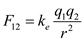Here,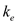is the Coulomb's constant,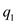is the charge of the particle 1,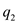is the charge of the particle 2, and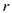is the distance between the two particles.
Substitute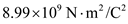for,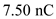for,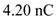for, and 1.80 m for r.Therefore, the magnitude of force between two charges is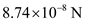.
(b)
The charges given in the problem are of same king. As the both the charges are of same sign, they must experience a repulsive force.

TagsExplain from an atomic viewpoint why charge is usually transferred by electrons.
Free
Essay

An atom consists of positively charged protons inside the nucleus and negatively charged electrons revolve round the nucleus in various circular orbits. Protons inside the nucleus are bound together by nuclear forces. The electrons in the atom are bound with the nucleus through electric interaction. Since the nuclear forces are stronger than the electrostatic forces, electrons are more mobile than protons and are more easily removed from atoms than the protons

TagsA charged particle A exerts a force of 2.62 N to the right on charged particle B when the particles are 13.7 mm apart. Particle B moves straight away from A to make the distance between them 17.7 mm. What vector force does particle B then exert on A
Essay
TagsA person is placed in a large, hollow metallic sphere that is insulated from ground. If a large charge is placed on the sphere, will the person be harmed upon touching the inside of the sphere
Essay
TagsTwo metal balls A and B of negligible radius are floating at rest on Space Station Freedom between two metal bulkheads, connected by a taut nonconducting thread of length 2.00 m. Ball A carries charge q , and ball B carries charge 2 q. Each ball is 1.00 m away from a bulkhead. (a) If the tension in the string is 2.50 N, what is the magnitude of q (b) What happens to the system as time passes Explain.
Essay
TagsWhy must hospital personnel wear special conducting shoes while working around oxygen in an operating room What might happen if the personnel wore shoes with rubber soles
Essay
TagsA small sphere of mass m = 7.50 g and charge q 1 = 32.0 nC is attached to the end of a string and hangs vertically as in Figure P15.4. A second charge of equal mass and charge q 2 = 258.0 nC is located below the first charge a distance d = 2.00 cm below the first charge as in Figure P15.4. (a) Find the tension in the string. (b) If the string can withstand a maximum tension of 0.180 N, what is the smallest value d can have before the string breaks
Essay
Tags(a) Would life be different if the electron were positively charged and the proton were negatively charged (b) Does the choice of signs have any bearing on physical and chemical interactions Explain your answers.
Essay
TagsThe nucleus of 8 Be, which consists of 4 protons and 4 neutrons, is very unstable and spontaneously breaks into two alpha particles (helium nuclei, each consisting of 2 protons and 2 neutrons). (a) What is the force between the two alpha particles when they are 5.00 × 10 15 m apart, and (b) what is the initial magnitude of the acceleration of the alpha particles due to this force Note that the mass of an alpha particle is 4.002 6 u.
Essay
TagsIf a suspended object A is attracted to a charged object B , can we conclude that A is charged Explain.
Essay
TagsA molecule of DNA (deoxyribonucleic acid) is 2.17 mm long. The ends of the molecule become singly ionized: negative on one end, positive on the other. The helical molecule acts like a spring and compresses 1.00% upon becoming charged. Determine the effective spring constant of the molecule.
Essay
TagsExplain how a positively charged object can be used to leave another metallic object with a net negative charge. Discuss the motion of charges during the process.
Essay
TagsA small sphere of charge 0.800 C hangs from the end of a spring as in Figure P15.7a. When another small sphere of charge 20.600 C is held beneath the first sphere as in Figure P15.7b, the spring stretches by d = 3.50 cm from its original length and reaches a new equilibrium position with a separation between the charges of r = 5.00 cm. What is the force constant of the spring
Essay
TagsConsider point A in Figure CQ15.8 located an arbitrary distance from two point charges in otherwise empty space. (a) Is it possible for an electric field to exist at point A in empty space (b) Does charge exist at this point (c) Does a force exist at this point
Essay
TagsFour point charges are at the corners of a square of side a as shown in Figure P15.8. Determine the magnitude and direction of the resultant electric force on q , with k e , q , and a left in symbolic form.
Essay
TagsA student stands on a thick piece of insulating material, places her hand on top of a Van de Graaffgenerator, and then turns on the generator. Does she receive a shock
Essay
TagsTwo small identical conducting spheres are placed with their centers 0.30 m apart. One is given a charge of 12 × 10 9 C, the other a charge of 218 × 10 9 C. (a) Find the electrostatic force exerted on one sphere by the other. (b) The spheres are connected by a conducting wire. Find the electrostatic force between the two after equilibrium is reached, where both spheres have the same charge.
Essay